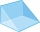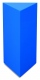# Volume + right triangle - math problems

#### Number of problems found: 147

• Conical areaA right angled triangle has sides a=12 and b=19 in right angle. The hypotenuse is c. If the triangle rotates on the c side as axis, find the volume and surface area of conical area created by this rotation.
• RotationThe right triangle with legs 11 cm and 18 cm rotate around the longer leg. Calculate the volume and surface area of the formed cone.
• Triangular prismThe perpendicular triangular prism is a right triangle with a 5 cm leg. The content of the largest wall of the prism is 130 cm2 and the body height is 10 cm. Calculate the body volume.
• CalculateCalculate the surface and volume of the cone that results from the rotation of the right triangle ABC with the squares 6 cm and 9 cm long around the shorter squeegee.
• Triangular prismCalculate the surface area and volume of a triangular prism, base right triangle if a = 3 cm, b = 4 cm, c = 5 cm, and height of prism h=12 cm.
• PrismThe base of a perpendicular triangular prism is a right triangle with legs 4.5 cm and 6 cm long. What is the surface of the prism, if its volume is 54 cubic centimeters?
• Vertical prismThe base of the vertical prism is a right triangle with leg a = 5 cm and a hypotenuse c = 13 cm. The height of the prism is equal to the circumference of the base. Calculate the surface area and volume of the prism
• Prism heightWhat is the height of the prism with the base of a right triangle with 6 cm and 9 cm? The diaphragm is 10.8 cm long. The volume of the prism is 58 cm3. Calculate its surface.
• Triangular prismThe base of the perpendicular triangular prism is a right triangle with a leg length of 5 cm. The content area of the largest sidewall of its surface is 130 cm², and the height of the body is 10 cm. Calculate its volume.
• Axial sectionThe axial section of the cone is an equilateral triangle with area 168 cm2. Calculate the volume of the cone.
• The volumeThe volume of the cone is 94.2dm³, the radius of the base is 6 dm Calculate the surface of the cone.
• Perpendicular prismCalculate the volume of the perpendicular prism if its height is 60.8 cm and the base is a rectangular triangle with 40.4 cm and 43 cm legs.
• TetrahedronCalculate height and volume of a regular tetrahedron whose edge has a length 4 cm.
• Surface and volumeFind the surface and volume of the rotating cone if the circumference of its base is 62.8 m and the side is 25 m long.
• Triangular pyramidWhat is the volume of a regular triangular pyramid with a side 3 cm long?
• Maximum of volumeThe shell of the cone is formed by winding a circular section with a radius of 1. For what central angle of a given circular section will the volume of the resulting cone be maximum?
• A concrete pedestalA concrete pedestal has a shape of a right circular cone having a height of 2.5 feet. The diameter of the upper and lower bases are 3 feet and 5 feet, respectively. Determine the lateral surface area, total surface area, and the volume of the pedestal.
• ConeCalculate the volume of the rotating cone with a base radius 26.3 cm and a side 38.4 cm long.
• Cube diagonalsCalculate the length of the side and the diagonals of the cube with a volume of 27 cm3.
• Tetrahedral pyramidCalculate the surface S and the volume V of a regular tetrahedral pyramid with the base side a = 5 m and a body height of 14 m.

Do you have an interesting mathematical word problem that you can't solve it? Submit a math problem, and we can try to solve it.

We will send a solution to your e-mail address. Solved examples are also published here. Please enter the e-mail correctly and check whether you don't have a full mailbox.

Please do not submit problems from current active competitions such as Mathematical Olympiad, correspondence seminars etc...

Tip: Our volume units converter will help you with the conversion of volume units. See also our right triangle calculator. Volume - math word problems. Right triangle Problems.